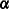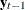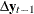The VARMAX Procedure

Bayesian Vector Error Correction Model

Bayesian inference on a cointegrated system begins by using the priors ofobtained from the VECM(p) form. Bayesian vector error correction models can improve forecast accuracy for cointegrated processes.

The following statements fit a BVECM(2) form to the simulated data. You specify both the PRIOR= and ECM= options for the Bayesian vector error correction model. The VARMAX procedure output is shown in Figure 35.17.

```/*--- Bayesian Vector Error-Correction Model ---*/

proc varmax data=simul2;
model y1 y2 / p=2 noint
prior=( lambda=0.5 theta=0.2 )
ecm=( rank=1 normalize=y1 )
print=(estimates);
run;
```

Figure 35.17 shows the model type fitted to the data, the estimates of the adjustment coefficient (), the parameter estimates in terms of lag one coefficients (), and lag one first differenced coefficients ().

Figure 35.17: Parameter Estimates for the BVECM(2) Form

The VARMAX Procedure

Type of Model BVECM(2) Maximum Likelihood Estimation 1 0.5 0.2

Alpha
Variable 1
y1 -0.34392
y2 0.16659

Parameter Alpha * Beta' Estimates
Variable y1 y2
y1 -0.34392 0.67262
y2 0.16659 -0.32581

AR Coefficients of Differenced Lag
DIF Lag Variable y1 y2
1 y1 -0.80070 -0.59320
y2 0.33417 -0.53480